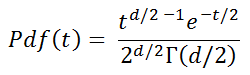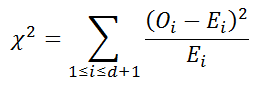# Chi-Square Test Calculator

The chi-square distribution with d degrees of freedom gives distribution of the sum of the squares of d normally distributed random variables. Its probability density function isThe chi-square distribution is used in hypothesis testing to determine the likelihood of the null hypothesis--that the observations are consistent with a theoretically assumed distribution. The test statistic is χ² iswhere Oi is the number of observations in group i, and Ei is the expected number in group i. The degrees of freedom is usually 1 less than the number of observations. In practical applications, researchers often reject the null hypothesis if the probability is less than a given threshold, such as 0.05 or 0.01.

### Chi-Square Test Calculator

 Observed Expected Use the mean number of observations as the expected values. Do not fill out "Expected" row. Enter expected values manually. The sum of the expected values must equal the sum of the observations.

Use the calculator above to compute the chi-square test statistic and probability for a set of observations. (You can also use a chi-square table.)

### Example

A researcher operates under the assumption that 40% of people in a given area (between the ages of 18 and 65) are employed full-time outside the home, 35% are employed part-time outside the home, and 25% fall into other categories. This assumption is the null hypothesis. He samples 320 people and comes up with the following observed data:

Full-time outside the home: 113
Part-time outside the home: 117
Other: 90

The expected data is

Full-time outside the home: 320(0.40) = 128
Part-time outside the home: 320(0.35) = 112
Other: 320(0.25) = 80

The degrees of freedom is 2 and the chi-square value is

(113-128)²/128 + (117-112)²/112 + (90-80)²/80 = 3.23102

The probability of obtaining these observations, given a theoretical distribution of 40%-35%-25%, is 0.198789. This is not low enough to reject the hypothesis; he has no reason to assume that the underlying distribution is not 40%-35%-25%.